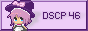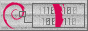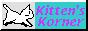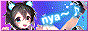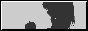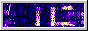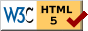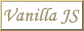dscp46, aka isithran

# Touchscreen calibration data converter

If this is not the first time you're not running a calibration, make sure to remove former calibration data, and rebstart your computer. Failure to do so will give wrong results.

First, to get the calibration data, run xinput_calibrator -v in a terminal, and let yourself be guided by the instructions.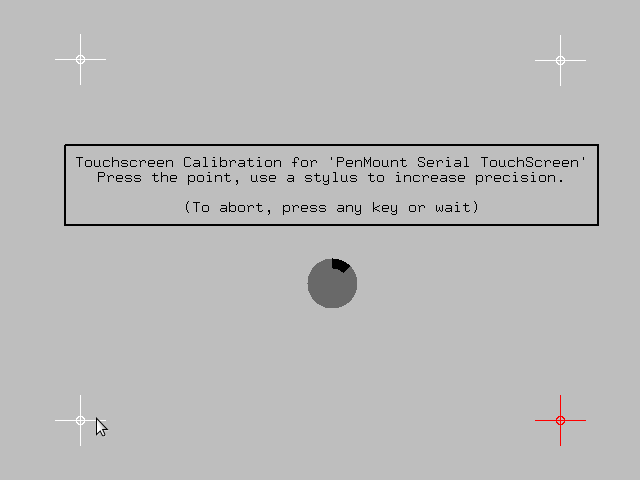Then, get your screen resolution. You can find it by running xrandr | grep '[^0-9]+' in a terminal.

One the calibration is completed, search the 4 following lines in the terminal.

DEBUG: Adding click 0 (X=174, Y=128)
DEBUG: Adding click 1 (X=1129, Y=137)
DEBUG: Adding click 2 (X=177, Y=893)
DEBUG: Adding click 3 (X=1121, Y=898)

Copy the corresponding values below:

Click 0:
X= – Y=
Click 1:
X= – Y=
Click 2:
X= – Y=
Click 3:
X= – Y=
Screen width:
pixels
Screen height:
pixels

# How it's calculated

As specified by the Wayland Documentation, libinput can rely on a device-specific coordinate transformation matrix $$A_d$$ that needs to be associated through udev.

The coordinates of four points $$\{x_0,y_0\} ... \{x_3,y_3\}$$ situated near each corner of the screen are needed to get actual coordinates. To facilitate the calibration, xinput_calibrator puts those points away from the border with a margin of $$\frac{1}{8}$$ of the screen size, to avoid issues with dead corners.

The last required values are the screen width $$S_w$$ and the screen height $$S_h$$. To get our parameters, we thus need to solve the following:

$$A_d = \begin{bmatrix} \frac{1}{8} & \frac{7}{8} & \frac{1}{8} & \frac{7}{8} \\ \frac{1}{8} & \frac{1}{8} & \frac{7}{8} & \frac{7}{8} \\ 1 & 1 & 1 & 1 \end{bmatrix} \cdot \begin{bmatrix} \frac{x_0}{S_w} & \frac{x_1}{S_w} & \frac{x_2}{S_w} & \frac{x_3}{S_w} \\ \frac{y_0}{S_h} & \frac{y_1}{S_h} & \frac{y_2}{S_h} & \frac{y_3}{S_h} \\ 1 & 1 & 1 & 1 \end{bmatrix}^{+}$$

The operation represented by the $$+$$ symbol is the Moore-Penrose inverse (aka pseudo-inverse).

In our case, due to the structure of our coordinates, we can infer the matrix to be full row rank. Thus, we can compute the pseudo-inverse using the QR method as follow: $$A^+ = A^T \cdot(A \cdot A^T)^{-1}$$, $$A^T$$ being the transposition, and $$A^{-1}$$ being the general inverse.

Once we've got $$A_d$$, we need to extract from it the elements $$a_{11}$$, $$a_{13}$$, $$a_{22}$$ and $$a_{23}$$. The other elements are approximately $$0$$ (except for $$a_{33} = 1$$), and thus don't bear any significance.

### References

: “Static device configuration via udev,” wayland.freedesktop.org. https://wayland.freedesktop.org/libinput/doc/latest/device-configuration-via-udev.html#static-device-configuration-via-udev (accessed Sep. 24, 2023).